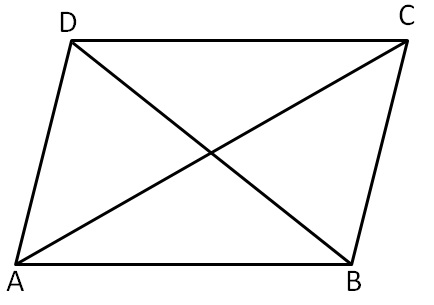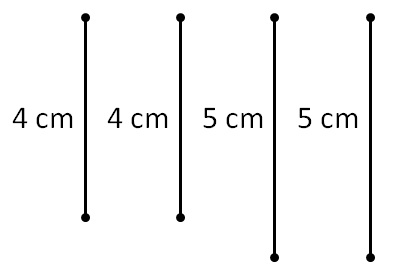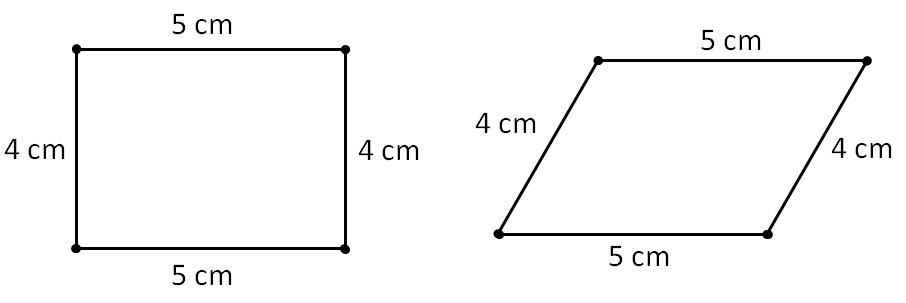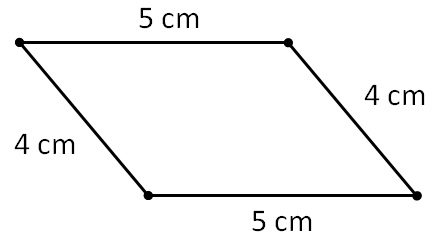Practical Geometry
Concept wise

• 4 sides
• 4 angles
• 2 diagonalsTo draw quadrilateral, we need any 5 measurements (not 4)

• 4 sides & 1 diagonal
• 3 sides & 2 diagonals
• Two adjacent sides and three angles
• 3 sides and two included angles
• When other special properties are known. (Like square’s side)

Let’s look at them one by one

### Why does Quadrilateral need 5 measurements? Why not 4?

We know that to draw a triangle,

we need 3 measurements – 3 sides, 2 angle 1 side etc.

So, for Quadrilateral, we should need 4 measurements

Eg: 4 sides

Let’s draw a quadrilateral with 4 sides as 5 cm, 4 cm, 5 cm, 4 cm

To construct it, let’s assume we have 4 sticks of sizes - 5 cm, 4 cm, 5 cm, 4 cmLet’s connect the lines to make a quadrilateral

We can join the lines likeOr likeThus,

we get different quadrilaterals when we have 4 measurements.

we need 5 measurements

In this chapter, we will learn how to make a quadrilateral when

• 4 sides and diagonal given
• 2 Diagonals and 3 sides given
• 2 Adjacent Sides and 3 angles given
• 3 Sides and 2 angles given
• Constructing Special Quadrilaterals like Square, Rectangle, Parallelogram, Rhombus

Let’s learn them one by one

Learn in your speed, with individual attention - Teachoo Maths 1-on-1 Class